•
•
•
•
•
•
•
•

# PROBLEMS BASED ON AGES [SHORT-TRICKS]

Today we are going to talk about some important arithmetic formulae on PROBLEM-BASED ON AGES, which is very important for types of competitive examination. Students are advised to learn these formulae and keep practicing with them.SOME IMPORTANT SUTRAS

1). If t yrs earlier, the age of the father is x times the age of his son. At present time, the age of the father is y times the age of his son. Then their present age –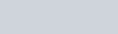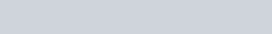2). If the present age of the father is x time the age of his son, t years later, the age of father become y times the age of his son, then their present ages-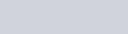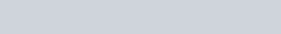3). If t1 yrs earlier, the age of the father is x times the age of his son. and hence t2 years later, the age of the father is y times the age of his son. Then their present age-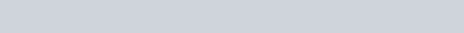4). If the ratio of the present age of A and B is x:y, and m years earlier, their ratio is a:b, then the equation of solution is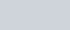5). If the ratio of the present age of A and B is x:y, and m years laters, their ratio is a:b, then the equation of solution is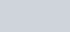6). If m years earlier, the ratio of the age of A and B is x:y and n years later, their ratio are a:b, then the equation of solution is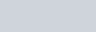7). If the ratio of age of A and B is a:b and the sum of their ages is S, their ages are-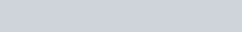8). If M is as smaller than N, as he is greater than P, and the sum of ages of N and P is S, such that P< M<N, then the age of M is =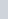This chapter contains a limited no of formulae, the best way to deal with such types of questions is that you have to learn to make equations by given data in one or two variables and solve them by proper methods.